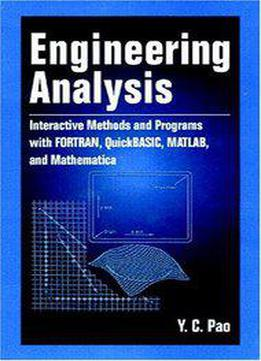# Engineering Analysis by Yen-Ching Pao / 1998 / English / PDF

This book provides a concise introduction to numerical concepts in engineering analysis, using FORTRAN, QuickBASIC, MATLAB, and Mathematica to illustrate the examples. Discussions include: matrix algebra and analysis solution of matrix equations methods of curve fit methods for finding the roots of polynomials and transcendental equations finite differences and methods for interpolation and numerical differentiation numerical computation of single and double integrals numerical solution of ordinary differential equations

views: 1172